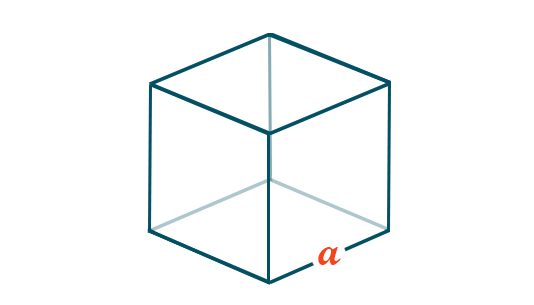# Volume of a Cube Formula

The volume of a cube is found by multiplying the value of the edge with itself three times. For instance, if the length of an edge is 5, the volume is 53. It should be noted that a cube has all edges of the same length and its volume is measured in cubic units. Here, we are going to discuss the cube formula to find the volume when the length of the edge/side is given with more solved examples.## Cube Formula to Find Volume

The Volume of a Cube Formula is,

The volume of a Cube, V = a3 Cubic units

Where,

• “a” is the side length of the cube.

### Solved Examples using the Volume of Cube Formula

Question 1:

What is the volume of a cube of side 5 cm?

Solution:

Given,

Side of the cube = a = 5 cm

We know that the volume of a Cube formula is V= a3

V= 53 cm3

∴ V = 125 cm3

Question 2:

What is the volume of a cube of side 15 cm?

Solution:

Given,

Side of the cube = a = 15 cm

The volume of a Cube V = a3

V = 153 cm3

∴ V = 3375 cm3

Question 3:

If the volume of a cube is 216 cm3, then what is the measure of the edge of a cube?

Solution:
Given,

Volume of a cube = V = 216 cm3
We know that, V = (side)3 or (edge)3
(edge)3 = 216 = 63
Therefore, edge of the cube = 6 cm